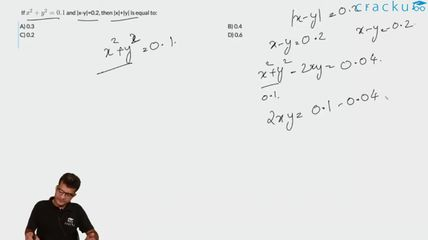Question 145

# If $$x^2 + y^2 = 0.1$$ and |x-y|=0.2, then |x|+|y| is equal to:

Solution

$$(x - y)^2 = x^2 + y^2 - 2xy$$

$$0.04 = 0.1 - 2xy => xy = 0.03$$

So, |xy| = 0.03

$$(|x| + |y|)^2 = x^2 + y^2 + 2|xy| = 0.1 + 0.06 = 0.16$$

So, |x|+|y| = 0.4

### View Video Solution